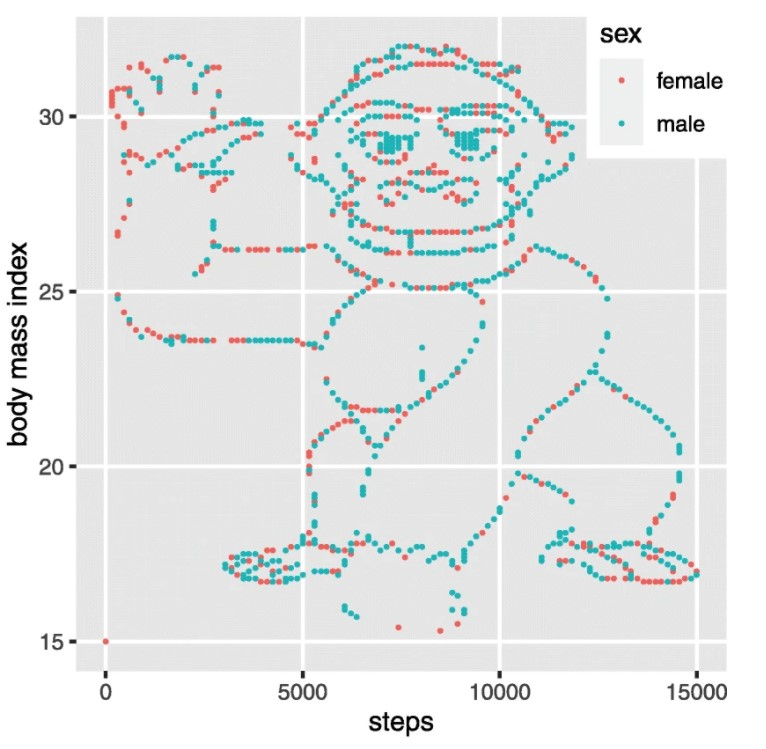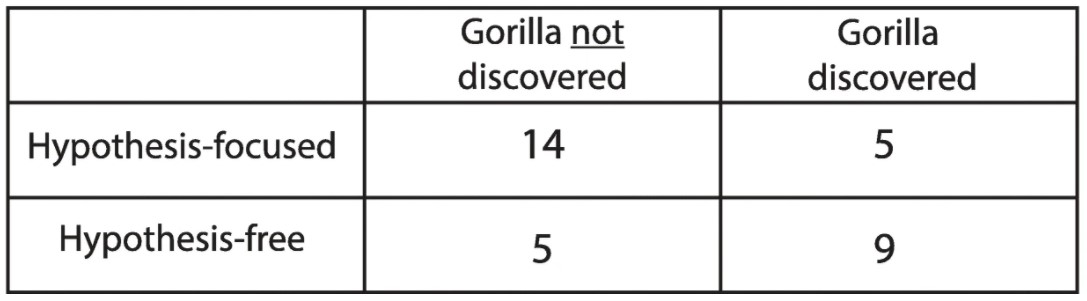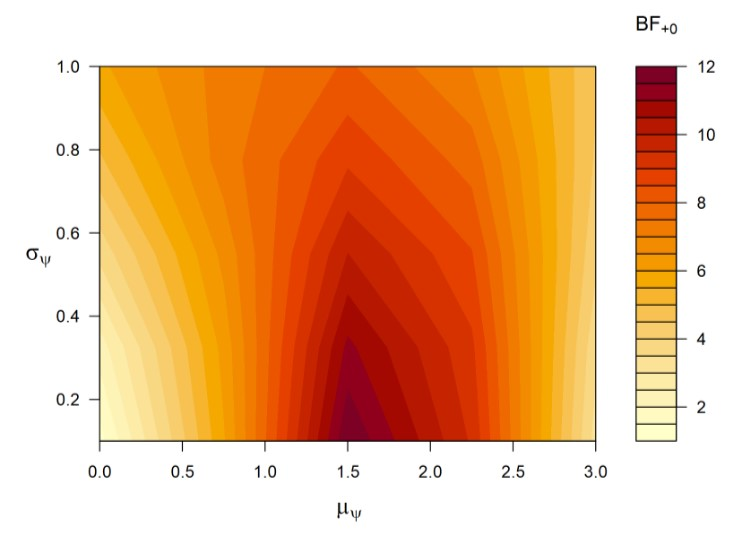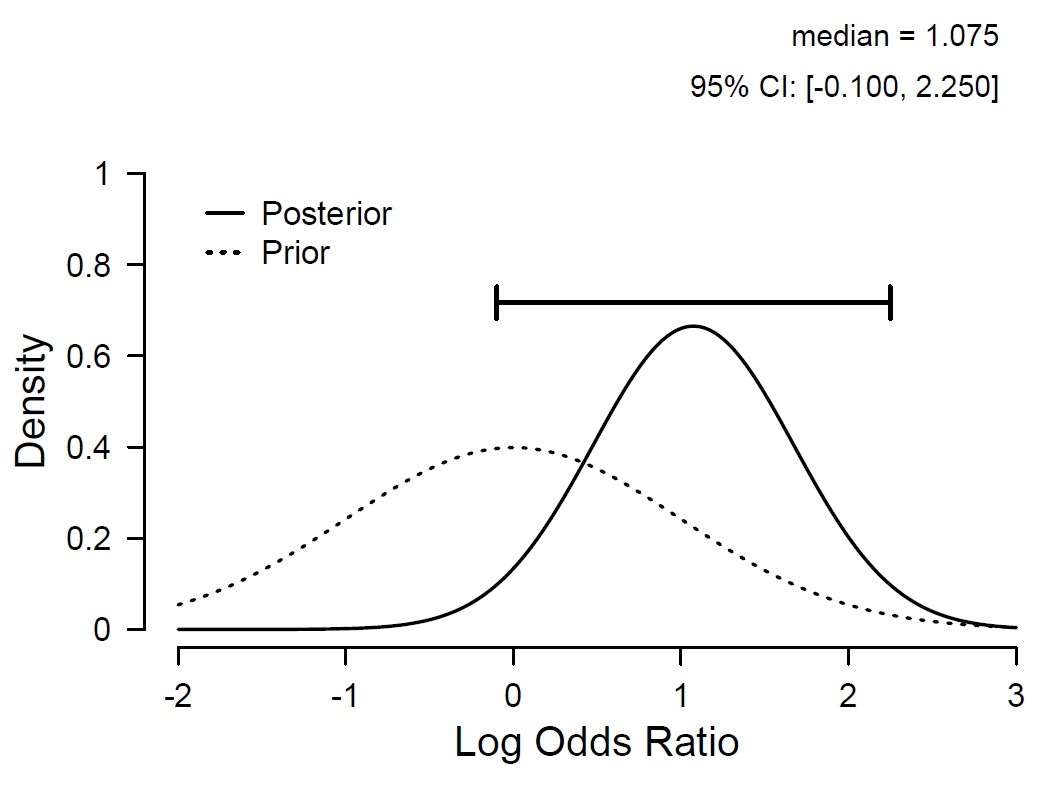# Redefine Statistical Significance XIX: Monkey Business

Background: the 2018 article “Redefine Statistical Significance” suggested that it is prudent to treat p-values just below .05 with a grain of salt, as such p-values provide only weak evidence against the null. Here we provide another empirical demonstration of this fact. Specifically, we examine the degree to which recently published data provide evidence for the claim that students who are given a specific hypothesis to test are less likely to discover that the scatterplot of the data shows a gorilla waving at them (p=0.034).

### Experiment and Results

In a recent experiment, Yanai & Lercher (2020; henceforth YL2020) constructed the statistical analogue of the famous Simons and Chabris demonstration of inattentional blindness , where a waving gorilla goes undetected when participants are instructed to count the number of passes with a basketball.

In YL2020, a total of 33 students were given a data set to analyze. They were told that it contained “the body mass index (BMI) of 1786 people, together with the number of steps each of them took on a particular day, in two files: one for men, one for women. (…) The students were placed into two groups. The students in the first group were asked to consider three specific hypotheses: (i) that there is a statistically significant difference in the average number of steps taken by men and women, (ii) that there is a negative correlation between the number of steps and the BMI for women, and (iii) that this correlation is positive for men. They were also asked if there was anything else they could conclude from the dataset. In the second, “hypothesis-free,” group, students were simply asked: What do you conclude from the dataset?”Figure 1. The artificial data set from YL2020 (available on https://osf.io/6y3cz/, courtesy of Yanai & Lercher via https://twitter.com/ItaiYanai/status/1324444744857038849). Figure from YL2020.

The data were constructed such that the scatterplot displays a waving gorilla, invalidating any correlational analysis (cf. Anscombe’s quartet). The question of interest was whether students in the hypothesis-focused group would miss the gorilla more often than students in the hypothesis-free group. And indeed, in the hypothesis-focused group, 14 out of 19 (74%) students missed the gorilla, whereas this happened only for 5 out of 14 (36%) students in the hypothesis-free group. This is a large difference in proportions, but, on the other hand, the data are only binary and the sample size is small. YL2020 reported that “students without a specific hypothesis were almost five times more likely to discover the gorilla when analyzing this dataset (odds ratio = 4.8, P = 0.034, N = 33, Fisher’s exact test (…)). At least in this setting, the hypothesis indeed turned out to be a significant liability.”Table 1. Results from the YL2020 experiment. Table from YL2020.

### Bayesian Reanalysis

We like the idea to construct a statistical version of the gorilla experiment, we believe that the authors’ hypothesis is plausible, and we also feel that the data go against the null hypothesis. However, the middling p=0.034 does make us skeptical about the degree to which these data provide evidence against the null. To check our intuition we now carry out a Bayesian comparison of two proportions using the A/B test proposed by Kass & Vaidyanathan (1992) and implemented in R and JASP (Gronau, Raj, & Wagenmakers, in press).

For a comparison of two proportions, the Kass & Vaidyanathan method amounts to logistic regression with “group” coded as a dummy predictor. Under the no-effect model H0, the log odds ratio equals ψ=0, whereas under the positive-effect model H+, ψ is assigned a positive-only normal prior N+(μ,σ), reflecting the fact that the hypothesis of interest (i.e., focusing students on the hypothesis makes them more likely to miss the gorilla, not less likely) is directional. A default analysis (i.e., μ=0, σ=1) reveals that the data are 5.88 times more likely under H+ than under H0. If the alternative hypothesis is specified to be bi-directional (i.e., two-sided), this evidence drops to 2.999, just in Jeffreys’s lowest evidence category of “not worth more than a bare mention”.

Returning to the directional hypothesis, we can show how the evidence changes with the values for μ and σ. A few keyboard strokes in JASP yield the following heatmap robustness plot:Figure 2. Robustness analysis for the results from YL2020.

This plot shows that the Bayes factor (i.e., the evidence) can exceed 10, but only when the prior is cherry-picked to have a location near the maximum likelihood estimate and a small variance. This kind of oracle prior is unrealistic. Realistic prior values for μ and σ generally produce Bayes factors lower than 6. Note that when both hypotheses are deemed equally likely a priori, a Bayes factor of 6 increases the prior plausibility for H+ from .50 to 6/7 = .86, leaving a non-negligible .14 for H0.

Finally, we can apply an estimation approach and estimate the log odds ratio using an unrestricted hypothesis. This yields the following “Prior and posterior” plot:Figure 3. Parameter estimation results for the data from YL2020.

Figure 3 shows that there exists considerable uncertainty concerning the size of the effect: it may be massive, but it may also be modest, or miniscule. Even negative values are not quite out of contention.

In sum, our Bayesian reanalysis showed that the evidence that the data provide is relatively modest. A p-value of .034 (“reject the null hypothesis; off with its head!”) is seen to correspond to one-sided Bayes factors of around 6. This does constitute evidence in favor of the alternative hypothesis, but its strength is modest and does not warrant a public execution of the null. We do have high hopes that an experiment with more participants will conclusively demonstrate this phenomenon.

### References

Benjamin, D. J. et al. (2018). Redefine statistical significance. Nature Human Behaviour, 2, 6-10.

Gronau, Q. F., Raj, K. N. A., & Wagenmakers, E.-J. (in press). Informed Bayesian inference for the A/B test. Journal of Statistical Software. Preprint: http://arxiv.org/abs/1905.02068

Kass, R. E., & Vaidyanathan, S. K. (1992). Approximate Bayes factors and orthogonal parameters, with application to testing equality of two binomial proportions. Journal of the Royal Statistical Society: Series B (Methodological), 54, 129-144.

Simons, D. J., & Chabris, C. F. (1999). Gorillas in our midst: Sustained inattentional blindness for dynamic events. Perception, 28, 1059-1074.

Yanai, I., & Lercher, M. (2020). A hypothesis is a liability. Genome Biology, 21:231.